Open in App
Not now

## Related Articles

• Write an Interview Experience

# Scatterplot using Seaborn in Python

• Difficulty Level : Basic
• Last Updated : 05 Nov, 2020

Seaborn is an amazing visualization library for statistical graphics plotting in Python. It provides beautiful default styles and color palettes to make statistical plots more attractive. It is built on the top of matplotlib library and also closely integrated into the data structures from pandas.

## Scatter Plot

Scatterplot can be used with several semantic groupings which can help to understand well in a graph. They can plot two-dimensional graphics that can be enhanced by mapping up to three additional variables while using the semantics of hue, size, and style parameters. All the parameter control visual semantic which are used to identify the different subsets. Using redundant semantics can be helpful for making graphics more accessible.

Syntax: seaborn.scatterplot(x=None, y=None, hue=None, style=None, size=None, data=None, palette=None, hue_order=None, hue_norm=None, sizes=None, size_order=None, size_norm=None, markers=True, style_order=None, x_bins=None, y_bins=None, units=None, estimator=None, ci=95, n_boot=1000, alpha=’auto’, x_jitter=None, y_jitter=None, legend=’brief’, ax=None, **kwargs)
Parameters:
x, y: Input data variables that should be numeric.

data: Dataframe where each column is a variable and each row is an observation.

size: Grouping variable that will produce points with different sizes.

style: Grouping variable that will produce points with different markers.

palette: Grouping variable that will produce points with different markers.

markers: Object determining how to draw the markers for different levels.

alpha: Proportional opacity of the points.

Returns: This method returns the Axes object with the plot drawn onto it.

## Creating a Scatter Plot

Let’s visualize of “fmri” dataset using seaborn.scatterplot() function. We will only use the x, y parameters of the function.

Code:

## Python3

 `import` `seaborn`  `seaborn.``set``(style``=``'whitegrid'``)``fmri ``=` `seaborn.load_dataset(``"fmri"``)` `seaborn.scatterplot(x``=``"timepoint"``,``                    ``y``=``"signal"``,``                    ``data``=``fmri)`

Output: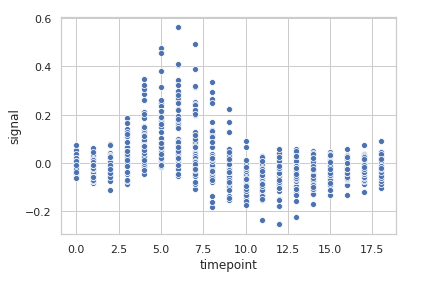Grouping data points on the basis of category, here as region and event.

## Python3

 `import` `seaborn`  `seaborn.``set``(style``=``'whitegrid'``)``fmri ``=` `seaborn.load_dataset(``"fmri"``)` `seaborn.scatterplot(x``=``"timepoint"``,``                    ``y``=``"signal"``,``                    ``hue``=``"region"``,``                    ``style``=``"event"``,``                    ``data``=``fmri)`

Output: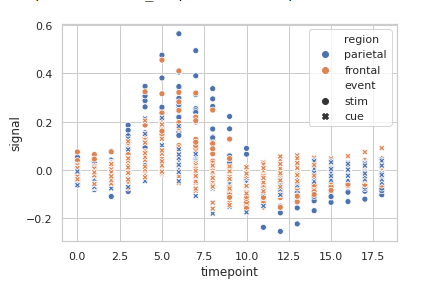Basic visualization of “tips” dataset using Scatterplot.

## Python3

 `import` `seaborn`  `seaborn.``set``(style``=``'whitegrid'``)``tip ``=` `seaborn.load_dataset(``'tips'``)` `seaborn.scatterplot(x``=``'day'``, y``=``'tip'``, data``=``tip)`

Output: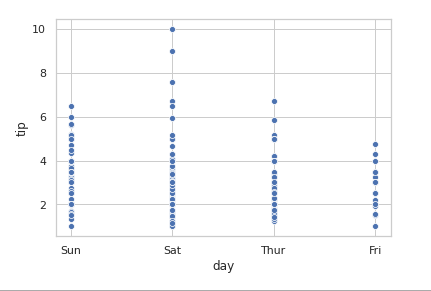## Grouping variables in Seaborn Scatter Plot with different attributes

The circle is used to represent the data point and the default marker here is a blue circle. In the above output, we are seeing the default output for the marker, but we can customize this blue circle with marker attributes.

## Python3

 `seaborn.scatterplot(x``=``'day'``, y``=``'tip'``, data``=` `tip, marker ``=` `'+'``)`

Output: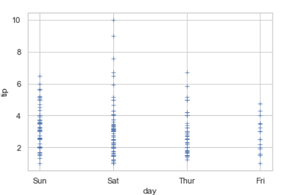It will produce data points with different colors. Hue can be used to group to multiple data variable and show the dependency of the passed data values are to be plotted.

Syntax: seaborn.scatterplot( x, y, data, hue)

## Python3

 `seaborn.scatterplot(x``=``'day'``, y``=``'tip'``, data``=``tip, hue``=``'time'``)`

Output: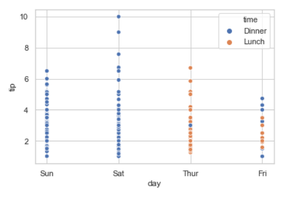In the above example, we can see how the tip and day bill is related to whether it was lunchtime or dinner time. The blue color has represented the Dinner and the orange color represents the Lunch.

Let’s check for a hue = ” day “

## Python3

 `seaborn.scatterplot(x``=``'day'``, y``=``'tip'``, data``=``tip, hue``=``'day'``)`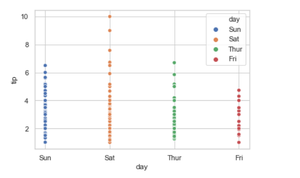Grouping variable that will produce points with different markers. Using style we can generate the scatter grouping variable that will produce points with different markers.

Syntax:

seaborn.scatterplot( x, y, data, style)

## Python3

 `seaborn.scatterplot(x``=``'day'``, y``=``'tip'``, data``=``tip, hue``=``"time"``, style``=``"time"``)`

Output: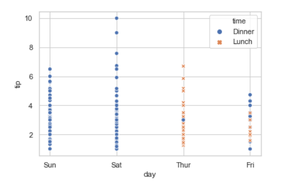Using the palette we can generate the point with different colors. In this below example we can see the palette can be responsible for a generate the scatter plot with different colormap values.

Syntax:

seaborn.scatterplot( x, y, data, palette=”color_name”)

## Python3

 `seaborn.scatterplot(x``=``'day'``, y``=``'tip'``, data``=``tip, hue``=``'time'``, palette``=``'pastel'``)`

Output: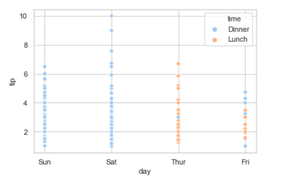Using size we can generate the point and we can produce points with different sizes.

Syntax:

seaborn.scatterplot( x, y, data, size)

## Python3

 `seaborn.scatterplot(x``=``'day'``, y``=``'tip'``, data``=``tip ,hue``=``'size'``, size ``=` `"size"``)`

Output: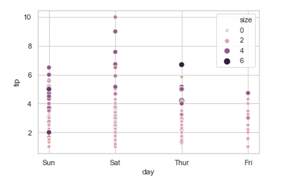.Using the legend parameter we can turn on (legend=full) and we can also turn off the legend using (legend = False).

If the legend is “brief”, numeric hue and size variables will be represented with a sample of evenly spaced values.

If the legend is “full”, every group will get an entry in the legend.

If False, no legend data is added and no legend is drawn.

Syntax: seaborn.scatterplot( x, y, data, legend=”brief)

## Python3

 `seaborn.scatterplot(x``=``'day'``, y``=``'tip'``, data``=``tip, hue``=``'day'``,``                    ``sizes``=``(``30``, ``200``), legend``=``'brief'``)`

Output: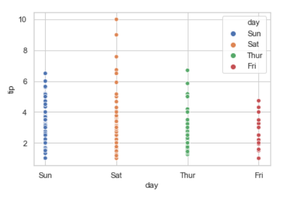Using alpha we can control proportional opacity of the points. We can decrease and increase the opacity.

Syntax: seaborn.scatterplot( x, y, data, alpha=”0.2″)

## Python3

 `seaborn.scatterplot(x``=``'day'``, y``=``'tip'``, data``=``tip, alpha ``=` `0.1``)`

Output: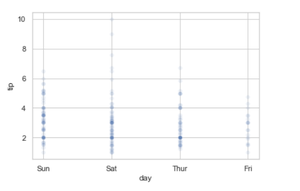My Personal Notes arrow_drop_up